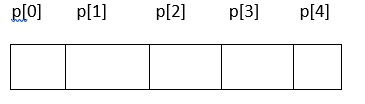# Explain the concept of Array of Pointer and Pointer to Pointer in C programming

## Array Of Pointers

Just like any other data type, we can also declare a pointer array.

## Declaration

datatype *pointername [size];

For example, int *p; //It represents an array of pointers that can hold 5 integer element addresses## Initialization

The ‘&’ is used for initialization

For example,

int a = {10,20,30};
int *p, i;
for (i=0; i<3; i++) (or) for (i=0; i<3,i++)
p[i] = &a[i];
p[i] = a+i;

## Accessing

Indirection operator (*) is used for accessing.

For example,

for (i=0, i<3; i++)
printf ("%d" *p[i]);

## Example

#include<stdio.h>
main (){
int a = {10,20,30};
int *p,i;
for (i=0; i<3; i++)
p[i] = &a[i]; //initializing base address of array
printf (elements of the array are”)
for (i=0; i<3; i++)
printf ("%d \t", *p[i]); //printing array of pointers
getch();
}

## Output

elements at the array are : 10 20 30

## Pointer to Pointer

Pointer to pointer is a variable that holds the address of another pointer.

## Declaration

datatype ** pointer_name;

For example, int **p; //p is a pointer to pointer

## Initialization

The ‘&’ is used for initialization.

Eg −

int a = 10;
int *p;
int **q;
p = &a;

## Accessing

Indirection operator (*) is used for accessing.

## Example

#include<stdio.h>
main (){
int a = 10;
int *p;
int **q;
p = &a;
q = &p;
printf("a =%d",a);
printf("a value through pointer = %d", *p);
printf("a value through pointer to pointer = %d", **q);
}

## Output

a=10
a value through pointer = 10
a value through pointer to pointer = 10